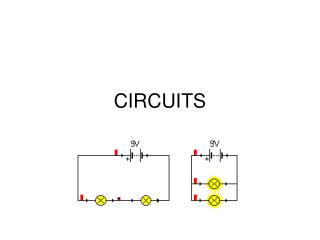DownloadDownload PresentationCIRCUITS

# CIRCUITS

Télécharger la présentation## CIRCUITS

- - - - - - - - - - - - - - - - - - - - - - - - - - - E N D - - - - - - - - - - - - - - - - - - - - - - - - - - -
##### Presentation Transcript

1. CIRCUITS

2. ELECTRON FLOW IN CIRCUITS • In metals, electric current is a flow of electrons. • How fast do these electrons move? • Electrons flow, at speeds on the order of centimeters per minute in DC circuits(battery). And in AC circuits the electrons don't really flow at all, instead they sit in place and vibrate. • "ELECTRICITY" MISCONCEPTIONS IN K-6 TEXTBOOKS - - -William J. Beaty • http://www.amasci.com/ele-edu.html

3. ELECTRON FLOW IN CIRCUITS • If the electrons are moving so slowly, why then does the light bulb turn on in such an instantaneous manner when we flip the switch????? • http://www.glenbrook.k12.il.us/gbssci/phys/Class/circuits/u9l4d.html

4. ELECTRON FLOW IN CIRCUITS • It's the energy in the circuit which flows fast, not the electrons. • Metals are always full of movable electrons. In a simple circuit, all of the wires are totally packed full of electrons all the time. And when a battery or generator pumps the electrons at one point in the circuit, electrons in the entire loop of the circuit are forced to flow, and energy spreads almost instantly throughout the entire circuit. This happens even though the electrons move very slowly. • "ELECTRICITY" MISCONCEPTIONS IN K-6 TEXTBOOKS - - -William J. Beaty • http://www.amasci.com/ele-edu.html

5. BICYCLE TIRE DEMO

6. Circuit diagrams are a pictorial way of showing circuits. Electricians and engineers draw circuit diagrams to help them design the actual circuits. Here is an example circuit diagram. This is the Ammeter symbol. This is the Voltmeter symbol. This is the resistor symbol. This is the battery symbol http://www.ndt-ed.org/EducationResources/HighSchool/Electricity/circuitdiagrams.htm

7. Series and parallelelectrical circuits are two basic ways of wiring components. The naming describes the method of attaching components A series circuit is one that has a single path for current flow through all of its elements. A parallel circuit is one that requires more than one path for current flow in order to reach all of the circuit elements. SERIES AND PARALLEL CIRCUITS http://en.wikipedia.org/wiki/Series_and_parallel_circuits

8. SERIES CIRCUIT • CURRENT -all elements in a series connection have equal currents. • VOLTAGE – Resistors with different values will have DIFFERENT voltages • All of the individual voltage drops across resistors should equal the voltage across the battery • Total Resistanceof Circuit - To find the total resistance of all the components, add together the individual resistances of each component http://en.wikipedia.org/wiki/Series_and_parallel_circuits

9. SERIES CIRCUIT • Total Resistance To find the current, use Ohm's law To find the voltage across any particular component

10. SERIES CIRCUITS • A series circuit is a circuit in which resistors are arranged in a chain, so the current has only one path to take. What happens if one device fails? • As more resistors are added the total resistance goes up. What does this do to current? http://physics.bu.edu/py106/notes/Circuits.html

11. PARALLEL CIRCUIT • CURRENT - Resistors with different values will have DIFFERENT current readings • VOLTAGE - Voltages across components in parallel with each other are the same • TOTAL RESITANCE OF CIRCUIT - To find the total resistance of all the components, add together the individual reciprocal of each resistance of each component, and take the reciprocal of the sum: http://en.wikipedia.org/wiki/Series_and_parallel_circuits

12. Total Resistance PARALLEL CIRCUIT To find the total current, I, use Ohm's Law To find individual currentIi= V/Ri To find VOLTAGE V= IiRi http://en.wikipedia.org/wiki/Series_and_parallel_circuits

13. PARALLEL • A parallel circuit is a circuit in which the resistors are arranged with their heads connected together, and their tails connected together. The current in a parallel circuit breaks up, with some flowing along each parallel branch and re-combining when the branches meet again. What happens is one device fails? • As resistors are added in parallel, total resistance goes down. What does this do to current? http://physics.bu.edu/py106/notes/Circuits.html